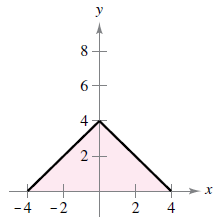Chapter 4.3, Problem 15E

Chapter
Section
Textbook Problem

Writing a Definite Integral In Exercises 13-22, write a definite integral that represents the area of the region. (Do not evaluate the integral.) f ( x ) = 4 − | x |To determine

To calculate: Definite integral representing the area of the provided region f(x)=4|x|.

Explanation

Given: f(x)=4|x| and, the region is:

Formula used:

On a closed interval [a,b], if f(x) is continuous and non-negative, then the area of the region bounded by the graph of f(x), the x-axis, and the vertical lines x=a and x=b can be determined using:

Area =abf(x)dx

Calculation:

From the provided figure, the region is bounded by the graph of

Still sussing out bartleby?

Check out a sample textbook solution.

See a sample solution

The Solution to Your Study Problems

Bartleby provides explanations to thousands of textbook problems written by our experts, many with advanced degrees!

Get Started

Domain Find the domain of the expression. 8. x4 + x3 + 9x

Precalculus: Mathematics for Calculus (Standalone Book)

let f(x) = x 1, g(x) = x+1, and h(x) = 2x3 1. Find the rule for each function. 12. gf

Applied Calculus for the Managerial, Life, and Social Sciences: A Brief Approach

In problems 7-10, replace each radical with a fractional exponent.Do not simplify. 7.

Mathematical Applications for the Management, Life, and Social Sciences